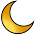## Friday, November 29, 2019

### DIY mass spectrometer measures potassium in dietary salt substitute

How to build and operate a simple mass spectrometer. Please ask questions in the comments, as always.

Scientific American article: https://www.scientificamerican.com/article/the-amateur-scientist-1970-07/  \$7.99 is a good value for the entire back issue in plain PDF format, and an example of a publisher being reasonable.   I'm happy to support this.

Original Dewdney paper: https://sci-hub.tw/https://doi.org/10.1119/1.1969211

https://en.wikipedia.org/wiki/Isotopes_of_potassium
https://en.wikipedia.org/wiki/Ionization_energy
https://en.wikipedia.org/wiki/Thermal_ionization

https://www.patreon.com/AppliedScience

1.What happened to the video?

2.I would expect that the changing voltage would also change the radius of ion path which would change that equation. Try it with an element that doesn't have many common stable isotopes and see if it really is an echo.

1.That is true, but that is how you scan over different m/z ratios. At any arbitrary voltage, only one m/z ratio will make it through the slit. (ideally)

3.I wondered if you could use a Z-axis input to color-code the slope polarity (assuming your scope would support pseudo-color on the Z-axis input). If your hypothesis is correct, the two peaks would be different colors. Alternatively, selectively remove the different slope polarities (blank on rising; blank on falling) using an external circuit to modify the X-axis input.

4.I was trying some numbers and my final thought is that your small peak is Tungsten 4+. Tungsten has oxidation levels of −4, −2, −1, 0, +1, +2, +3, +4, +5, and 6+. Where 4+ and 6+ are more common. (if I'm interpretating wikipedia correctly)

With the different isotopes of tungsten the voltages will (following R=0,018m; B=0,56T and q=4) vary between mass=181,95Ar -> 107,6V and mass=185.95Ar -> 105.3V

5.Hey Ben!
Here's a project for you that you may be interested in - designing a 'flow battery'. Kind of cool and a harvard prof recently recieved the enil prize (nobel prize equiv for energy) for his work in it.

https://faculty.chemistry.harvard.edu/gordon/news/gordon-and-aziz-accept-2019-eni-award